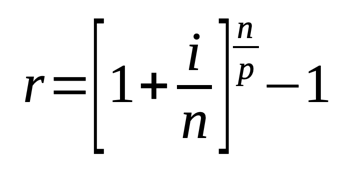# Simple Loan Calculator

Share:

This is a simple loan calculator used to calculate the periodic payment amount with some basic inputs.

This calculator does not have the options to include down payment, extra fee, balloon payment or changing the compounding period in loan calculation. For such details you can use our Advanced Loan Calculator.

Enter the required fields and press the Calculate Loan button to get the result.

*
*
\$
*
%
*
*
Years
Months
FromThis calculator is used to calculate a simple loan repayment amount for each payment period. It also calculates the total amount to be repaid, interest amount and the effective annual percentage rate. This calculator uses compounding of interest for interest calculation.

This calculator does not have the options to include down payment, extra fee and balloon payment. For such details you can use the Amortized Loan Calculator.

### Using Simple Loan Calculator

1. Select the Currency of your choice.
2. Enter the Loan Amount amount (principal amount).
3. Enter the yearly compound Interest Rate in percentage.
4. Select the Payment Period from the drop down.
5. Enter the Loan Term either in years and or months.
6. Finally, press the Calculate Loan button. The periodic payment amount, total interest amount, total payment amount, effective annual percentage rate (EAR), number of payments to do and the pay off date will be calculated and the result will be displayed.

## Loan

This calculator uses compounding of interest for interest calculation. Based on the compounding of interest, compounding period and the loan period we can find the repayment of loan amount per period.

### Formula To Find Loan Periodic Payments##### Where:
• A = Repayment of loan amount per period
• P = Loan Amount
• r = Interest Rate per period
• n = Total number of payments or total number of periods

#### Formula To Find Interest Rate per Period##### Where:
• r = Interest rate per period
• i = Nominal annual interest rate
• n = Number of compounding periods / year
• p = Number of payment period / year